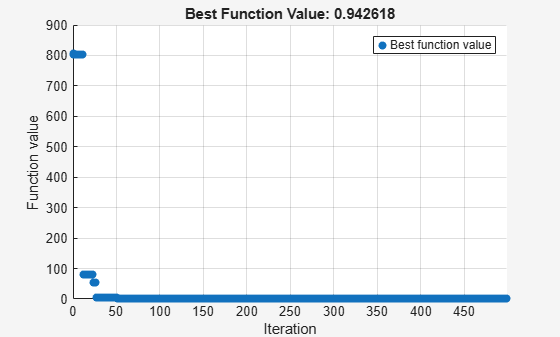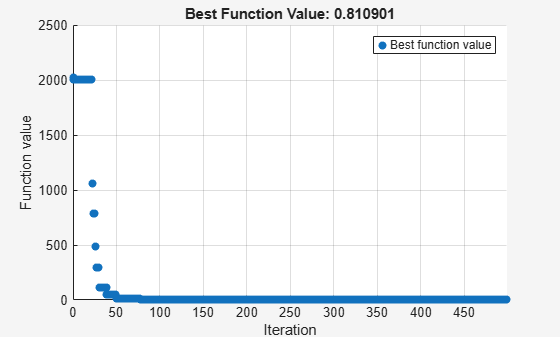# Fix Variables in `surrogateopt`

This example shows how to fix the values of some control variables, by removing them from an optimization. Although the easiest way to fix values is to set equal upper and lower bounds, some solvers do not allow equal bounds. However, `surrogateopt` handles equal bounds well by internally removing fixed variables from the problem before trying to optimize.

The `multirosenbrock` function accepts any even number of control variables. Its minimum value of 0 is attained at the point `[1,1,...,1,1]`. Set lower bounds of –1 and upper bounds of 5 for ten variables, and then set the first six upper and lower bounds equal to 1. This setting removes six variables from the problem, leaving a problem with four variables.

```lb = -1*ones(1,10); ub = 5*ones(1,10); lb(1:6) = 1; ub(1:6) = 1;```

Solve the problem.

```fun = @multirosenbrock; rng default % For reproducibility [x,fval,exitflag] = surrogateopt(fun,lb,ub)``````surrogateopt stopped because it exceeded the function evaluation limit set by 'options.MaxFunctionEvaluations'. ```
```x = 1×10 1.0000 1.0000 1.0000 1.0000 1.0000 1.0000 0.5195 0.2679 1.5180 2.3061 ```
```fval = 0.4999 ```
```exitflag = 0 ```

The solver returns a point close to the global minimum. Notice that the solver takes 500 function evaluations, which is the default value for a problem with 10 variables. The solver does not change this default value even when you fix some variables.

When you do not fix any variables, the solver does not reach a point near the global minimum.

```lb = -1*ones(1,10); ub = 5*ones(1,10); rng default % For reproducibility [x,fval,exitflag] = surrogateopt(fun,lb,ub)``````surrogateopt stopped because it exceeded the function evaluation limit set by 'options.MaxFunctionEvaluations'. ```
```x = 1×10 1.4639 2.1451 1.3645 1.8633 1.4170 2.0147 1.4286 2.0438 1.4343 2.0578 ```
```fval = 0.9003 ```
```exitflag = 0 ```